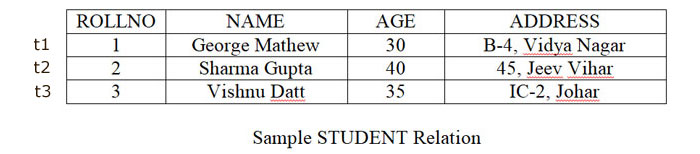#### Structure of Relational Databases

A relational database consists of a collection of tables, each of which is assigned a unique name. It has a structure similar to a two-dimensional table.

A relation consists of:
• Relational Schema
• Relation Instance

A Relational Schema specifies the relation’s name, its attributes and the domain of each attribute. If R is the name of a relation and A1, A2, …, An is a list of attributes representing R then R(A1, A2, …, An) is called a relational schema. Each attribute in this relational schema takes a value from some specific domain called Domain(Ai).
Eg:- PERSON(PERSON_ID : integer, NAME : string, AGE : integer, ADDRESS : string)
The degree of this relation is 4.
Total number of attributes in a relation denotes the degree of a relation.

A Relation Instance denoted as r is a collection of tuples for a given relational schema at a specified point of time. A relation state r of the relation schema R(A1, A2, …, An), denoted by r(R), is a set of n-tuples.

r = {t1, t2, …, tm}
where each m-tuple is an ordered list of n values.
t = < v1, v2, …, vn >
where each vi belongs to domain(Ai) or contains null values.In this instance m = 3 and n = 4.
In a relation, tuples are not inserted in any order. Ordering of tuples is not defined as a part of a relation definition. However, records may be organized later according to some attribute value in the storage system and such organization depends on the requirement of the underlying database application.